×

Search anything:

# Types of Neural Networks

#### Machine Learning (ML)Get this book -> Problems on Array: For Interviews and Competitive Programming

Now in the deep learning era, there are various types of neural networks present. Some of the popular Neural Networks ones are:

1. Convolutional Neural Network
2. Recurrent Neural Network
3. Residual Neural Network
4. Spiking Neural Network
5. Autoencoder
6. Other types like:
• Time Delay Neural Network (TDNN)
• Deep stacking network (DSN)
• Modular Neural Network
• Probabilistic Neural Network (PNN)
• Radial basis function Neural Network (RBF Network)

We will, first, review the basics of Neural Networks and then, understand each of the different types of Neural Network in detail.

## Neural Network basics

Neural networks is a type of network that basically mimics the functioning of the biological neurons in the human brain.
It is an interconnection of various layers. These layers are classified into three types:

1. Input Layer
2. Hidden Layer(s)
3. Output layer

The input layer provides the input to the neural network, as clear from the name. Hidden layer consist of multiple nodes that takes in inputs from the previous layer along with weigths(parameters). Then to these, bias value is added and these nodes with the help of specific activation functions provides an output.Finally the output layer shows the output.

To train a neural network following steps are required:

1. First obtain an output using Forward propagation(going from input layer to output layer).
2. Compare the obtained result with actual result i.e calculate the cost function.
3. Using backpropagation update the weights(using learning rate) and hence the cost function is minimized.
4. Finally obtain required result/model.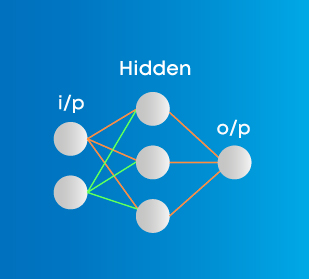Some important terms involved:

1. Activation function: It is basically a mathematical function such as sigmoid function, tanh function(hyperbolic tangent function) etc. The weighted sum( sum of all inputs multiplied by weights) is given to the activation function and based on
that the neuron(node) calculates some value(based on what function used) and the node then decides whether to pass the value or not. Each node can have different or same activation function depending on designer of the network.
2. Forward Propagation: Going from input layer to obtaining an output through the output layer is called forward propagation.
3. Cost function: It is a function that is used to measure the difference between calculated and actual output. Basically we need to minimize this cost function in order to obtain the most efficient results.
4. Backward propagation:It is basically opposite of forward propagation. Here we go from output to input layer updating the value of weights in order to minimize the cost function.
5. Learning rate: It is a hyper parameter that is used to update the weights(i.e by how much).

## Different types of Neural Networks

We shall now dive into the different types of Neural Networks.

# I. Convolutional Neural Network

It is the type of neural network that is mainly used to deal for analysis of images or videos. It searches for the important features and these features are used for classification. The prime application of CNNs is in image recognition and classification, natural language processing, recommender system etc.
Now there are certain steps involved in the CNN that helps in visual input analysis:

1. Convolution Operation: This process is used to obtain the feature map. What happens here is that the input image is taken in the form of matrix with pixel values. Here we make use of feature detector or filter that helps in reducing the size of the image but providing the important features. After applying the convolution operation, what we are left is called the feature map. Different filters are used to obtain different feature maps. To increase non-linearity in the image, Rectifier function can be applied to the feature map.

2. Pooling: This is another important step in convolutional neural network. This step helps to maintain spatial invariance or even deal with other kind of distortions in the process. This means that if we are trying to perform image recognition or something, like checking if the image contains a dog, there is a possibility that the image might not be straight(maybe tilted), or texture difference is there,
the object size in the image is small etc. So this should not let our model to provide incorrect output. This is what pooling is all about. There can be different types of pooling like min pooling, average pooling or max pooling. It helps to preserve the essential features. After applying max pooling(for example) on feature map, it uses the maximum values from the clusters. What we obtain is called pooled feature map. Here the size is reduced, features are preserved and distortions are dealt with.

3. Flattening: In this step, we basically convert our matrix(pooled feature map) into vector which then can be given as input to the further artificial neural network which performs the classification or identification.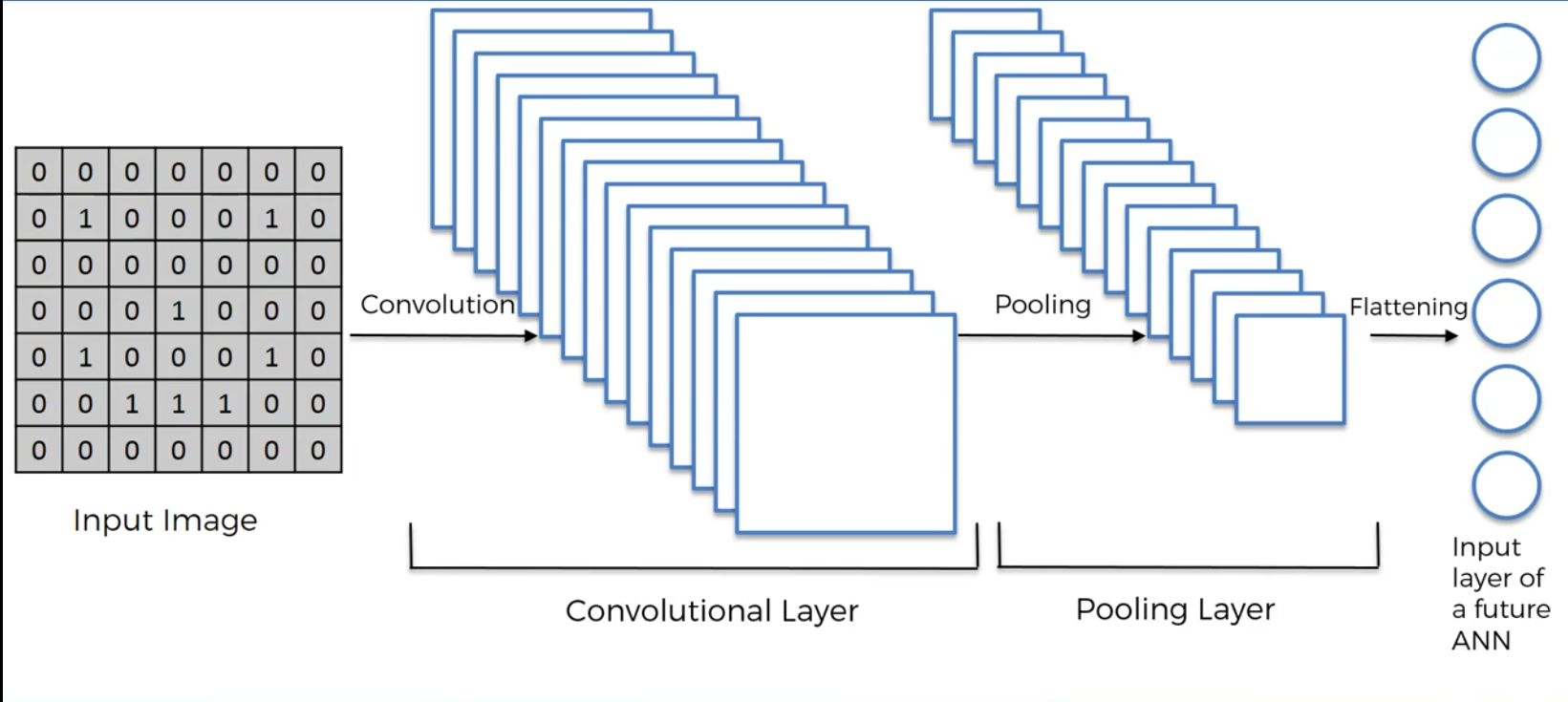# II. Recurrent Neural Network

The basic idea behind recurrent neural networks is saving previously calculated outputs for future prediction. What this means is that once functions are performed by the neurons, the output produced is stored for future. This makes each neuron act like a memory cell in performing computations. The outputs previously calculated are given as input for future processes. The best application of recurrent neural networks can be in the field of natural language processing(NLP). It is very useful in sentence completion, translation, sentiment analysis etc. If the predictions are wrong, learning rate is used to make small changes. One of the biggest challenges that RNN faces is the vanishing gradient problem. LSTM(Long Short Term Memory) provides solution for this problem.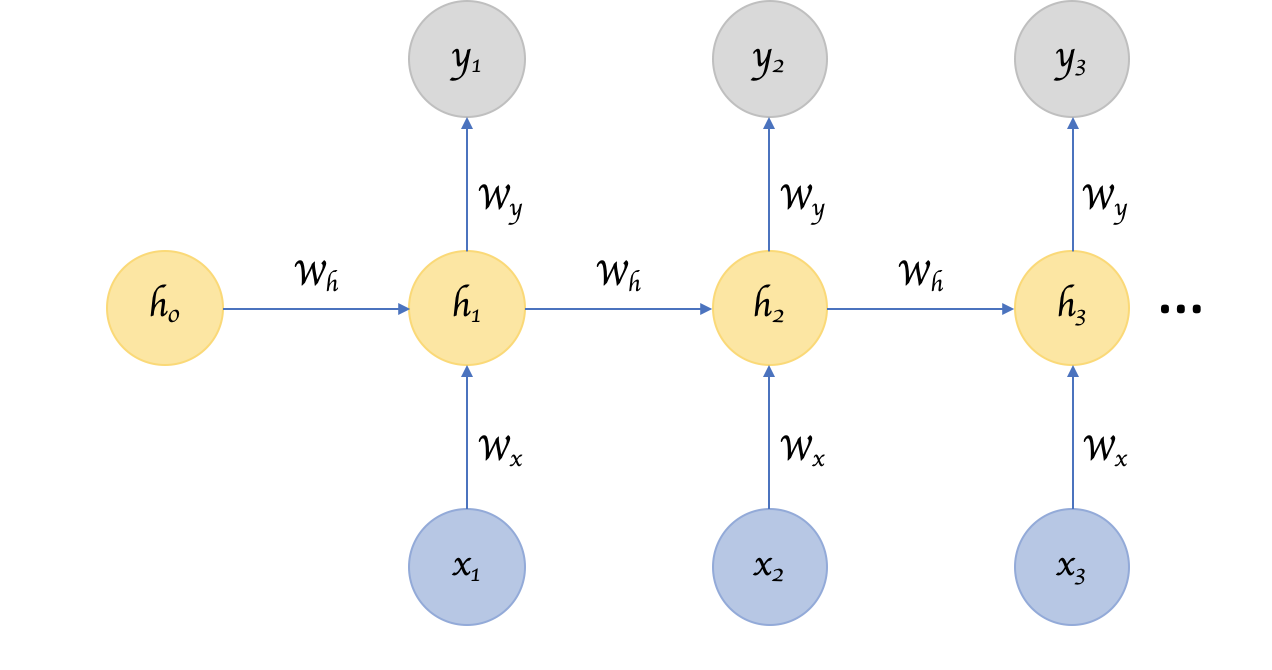# III. Residual Neural Networks

Residual neural network or also known as ResNet are the type of networks that are really helpful when it comes to dense network structure. In ResNet, the basic
modification that occurs is that the input given to some layer is passed to further layer. The following image demonstrates it: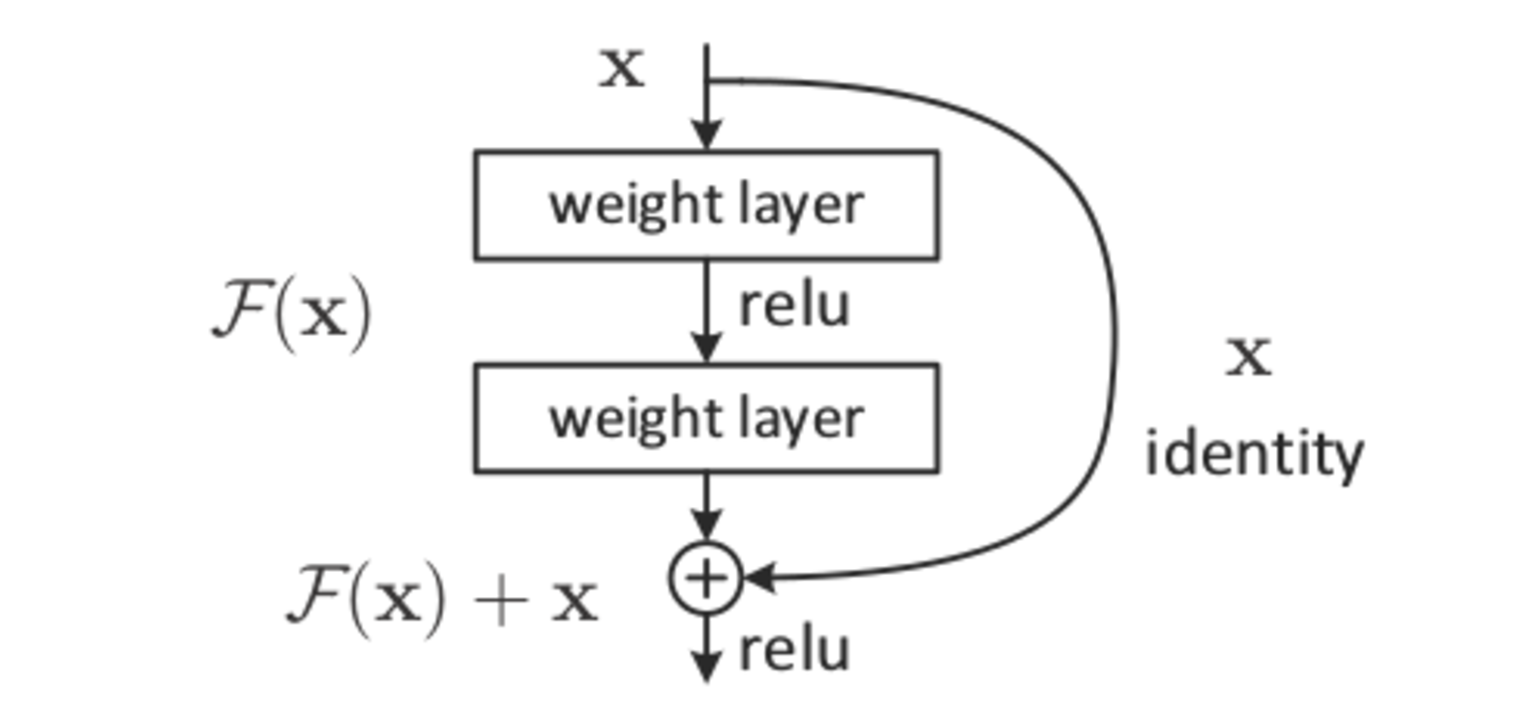These shortcuts that are introduced are called skip connections. These connections can skip from 1 layer to n number of layers based on requirement. If training time needs to be reduced then large numbers of layers can be skipped too. Consider a normal neural network(like CNN or simple ANN), if we increase the number of hidden layers i.e make our network more dense, it is supposed to increase the accuracy and reduce training error. But this practically does not happen and as we keep on increasing number of layers, after some point, instead of decreasing error rate slowly increases. This is where ResNets are useful as more the network is dense, error percentage keeps on decreasing.

# IV. Spiking Neural Networks

Spiking Neural Networks or SNNs are the next generation networks that are works more like biological network than the existing artificial neural networks. SNNs are quite
different from the neural networks that are currently used in the field of machine learning. The current neural networks works with continuous values. They take in input as continuous value and give out output as continuous values. SNN on the other hand works on spikes. Spikes are discrete events that take place at some time. Hence the network works when some event occurs. Now the question arises, how can we know when an event will occur? The answer to that question is differential equation. The occurrence of spikes are determined by differential equations.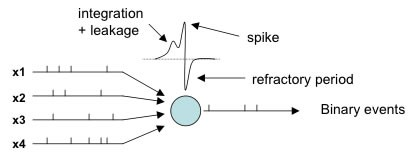In SNN, problem arises when it comes to training it. There is no efficient supervised training method for the SNNs that will help us getting better performance than the models of neural networks that currently are being used. As the spike trains are not differentiable, gradient descent cannot be used for spiking neural networks. Also the computation resources for training SNN is another parameter to worry about. Neuromorphic hardwares are required for that purpose as these hardwares are quite useful when it comes to perform biological brain like functioning. Also neuromorphic hardwares are quite helpful in simulating differential equations.
So currently SNNs are more like a theoretical concept as very less real life applications are there. But with proper advancements in technology, SNNs can turn out to be one of the most powerful concept in the field of Artificial intelligence.

# V. Autoencoder

An autoencoder neural network is an unsupervised learning algorithm in which the output is equal to the input. What it does is that it takes the input, encodes it and then try to reconstruct input as the output i.e output is like enhanced form of the input. Now in the autoencoder, certain phases are involved. First when the input is given, it reduces the dimension or compresses it and converts it into encoded representation. Now we have the input in the most reduced form as possible. Next comes the decoding part where the autoencoder reconstructs the input and presents it as the output. Reconstruction loss is calculated and minimized in order to obtain the most accurate model. Backpropagation is used to train the network and minimize
the loss.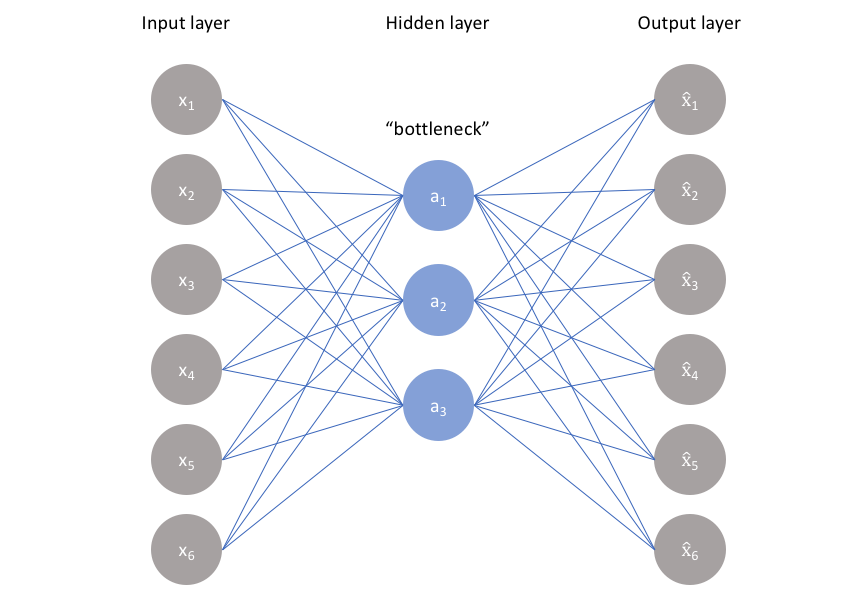There are various types of autoencoders like denoising autoencoder, sparse autoencoder, variational autoencoder and contractive autoencoder. Application of autoencoders can be image generation, image denoising, dimensionality reduction etc.

# Other types

There are many more types of Neural networks present in the current deep learning era like:

i. Time Delay Neural Network (TDNN): Time delay neural networks are quite similar to other neural networks with multiple layers and feed forward structure. The difference is that along with the inputs from previous layers, the nodes also have time-delayed outputs from these same layers. In TDNN, shift invariant classification is achieved. Applications of TDNN includes speech recognition, image recognition, video analysis etc.

ii. Deep stacking network (DSN): The structure of deep stacking networks is in hiearchy form. Each block of DSN can be train using supervised way without backpropagation for the entire blocks. This type of structure helps in better parallel learning. Tensor deep stacking network(T-DSN) is the extension of DSN.

iii. Modular Neural Network: In this type, modular concept is involved. Here several independent networks performs their functions and provide output. After that outputs of all modules are combined and processed to provide the final output.

iv. Probabilistic Neural Network (PNN): It is the type of supervised network that is mostly used for classification and pattern recognition. It involves calculating probability density function(PDF) of each class. Using PDF of each class, the class probability of a new input is estimated and Bayes’ rule is employed to allocate it to the class with the highest posterior probability.

v. Radial basis function Neural Network (RBF Network): This network works with the distance of points from the center. Gaussian functions are generally used in RBF networks.

With this, you have the complete knowledge of different types of Neural Networks.#### Apoorva Kandpal

I am a Deep learning enthusiast and am a student at SRM Institute of Science & Technology. I am able to handle multiple tasks on a daily basis. I am always energetic and eager to learn new skills

Types of Neural Networks